## Wednesday, June 12, 2019

### Aesthetic & functional updates to the function `ltt95` for visualizing a lineage-through-time plots for a set of trees

I just pushed some updates & additions (1, 2, 3, 4) to the phytools function `ltt95`.

`ltt95` (e.g., here) is a relatively simple function for drawing (1-α) × 100% CIs around a lineage through time plot. It has two different modes - one in which the mean or median & confidence intervals are computed in terms of the number of lineages at a given time (`method="lineages"`) and the other other in which the mean or median & confidence intervals are calculated for the amount of time that has elapsed, given a certain number of lineages (`method="times"`).

I mainly added some improved visualization - for instance, using a shaded polygon instead of simple dotted lines to demarcate the CIs. I'll be the first to admit that the style is unoriginal. I believe that the paleotree package produces a plot that is stylistically highly similar, if not the same. Nonetheless, the function has some potentially useful features so I thought it was worth doing. I also corrected the lower confidence bounds - previously it was one element too low making the CIs slightly too large.

Here's a demo using some phylogenies of primates:

``````library(phytools)
"http://www.phytools.org/TS2018/data/10kTrees_Primates.nex")
ltt95(primates,log=TRUE)
``````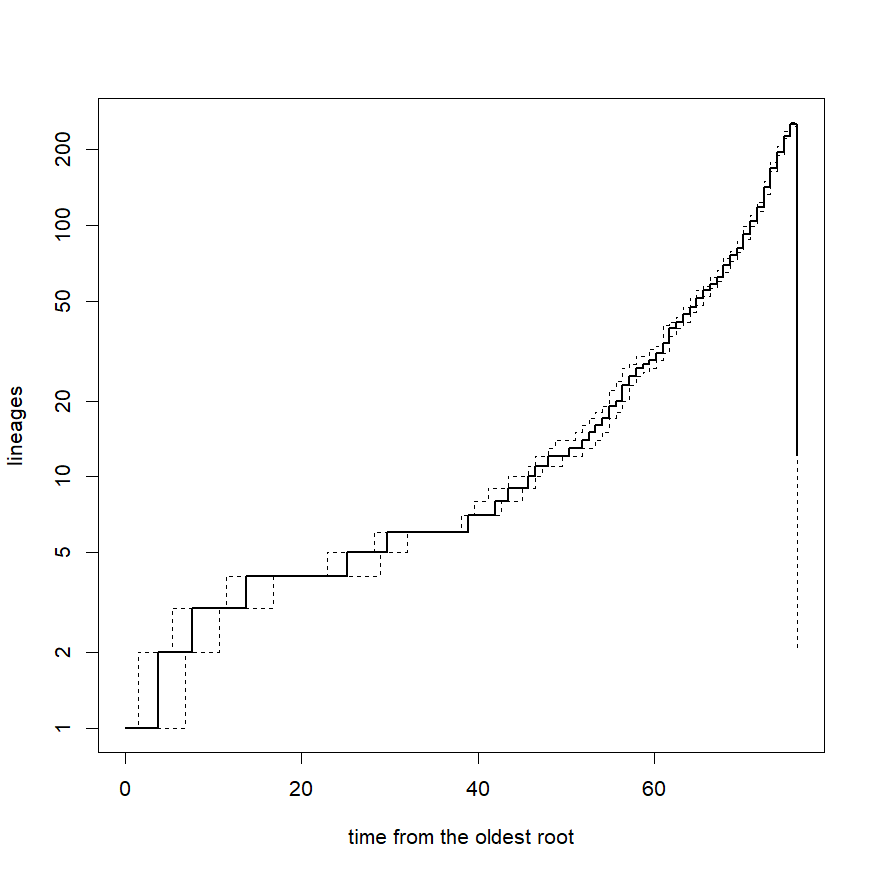The weird effect where the number of accumulated lineages drops off to zero at the end of the plot is a characteristic sign of some of the trees not being ultrametric to the precision of the machine. This is because `phytools::ltt` (used internally by `ltt95`) intentionally does not assume that the trees are ultrametric. In our case, they should be - and the small deviations that we see are merely due to rounding of the edge lengths when our trees were written to file.

To fix that, let's use `force.ultrametric` as follows:

``````primates<-lapply(primates,force.ultrametric)
class(primates)<-"multiPhylo"
``````

Now, let's proceed to re-compute our `"ltt95"` object, this time without plotting:

``````object<-ltt95(primates,log=TRUE,plot=FALSE)
object
``````
``````## Object of class "ltt95".
##   alpha: 0.05
##   method:    lineages
##   mode:      median
##   N(lineages):   273
``````
``````par(bty="l")
``````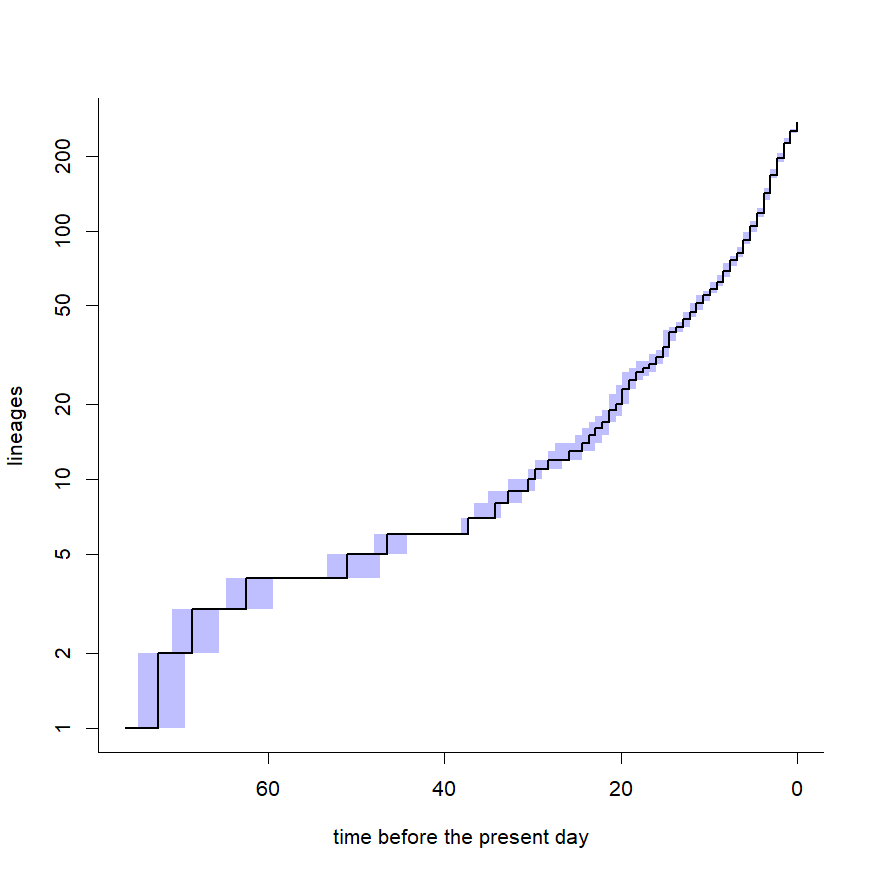That's cool. Now let's switch to `mode="times"`, just to see how it looks:

``````object<-ltt95(primates,log=TRUE,plot=FALSE,method="times")
object
``````
``````## Object of class "ltt95".
##   alpha: 0.05
##   method:    times
##   mode:      median
##   N(lineages):   273
``````
``````par(bty="l")
``````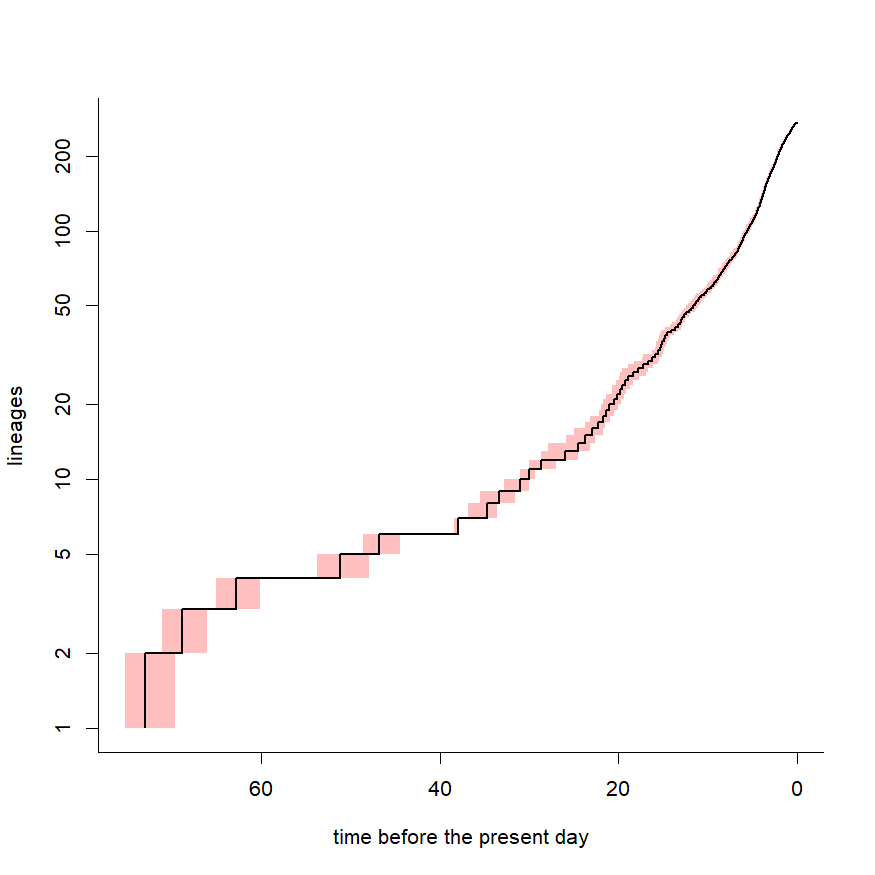Finally, here is what it looks like if we compute the average number of lineages at each time point, rather than the median. I'm also going to set α to 0.0 for the confidence intervals - essentially, I want my CI to include all trees in the sample:

``````object<-ltt95(primates,log=TRUE,plot=FALSE,mode="mean",alpha=0)
par(bty="l")
legend(x="topleft",c("mean number of lineages",
"total range of lineages across trees"),
lty=c(1,0),lwd=c(2,0),pch=c(NA,22),pt.cex=2.5,
pt.bg=c("black",rgb(0,0,1,0.25)),col=c("black","transparent"),
bty="n")
``````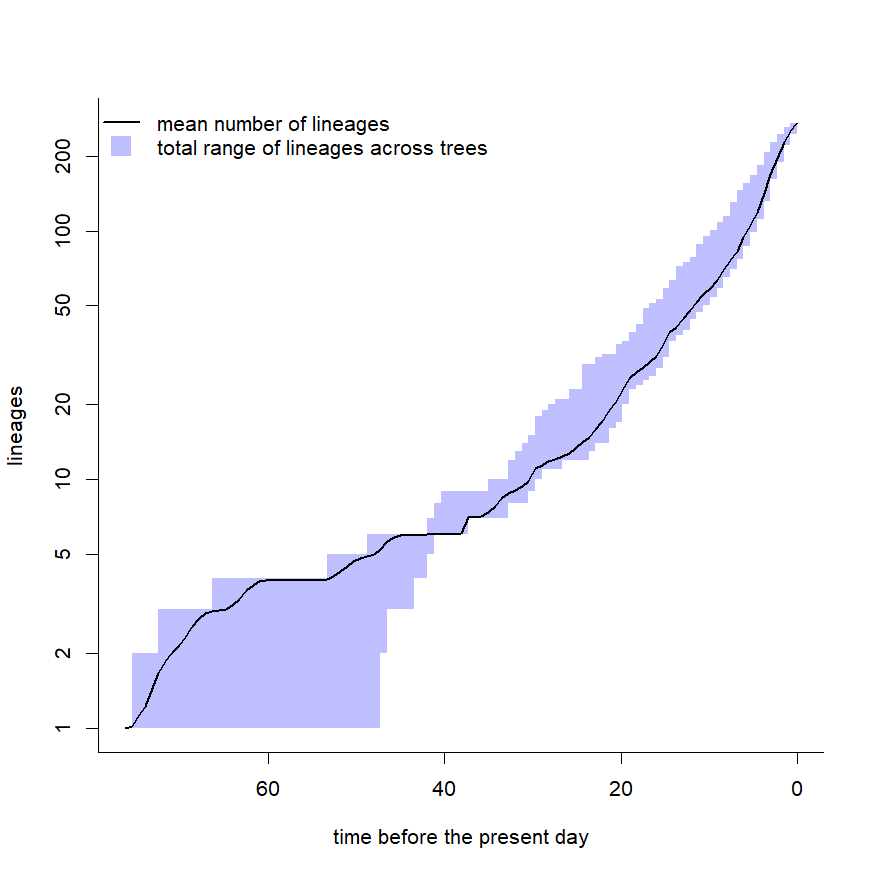Finally, here are all the LTTs plotted for comparison:

``````object<-ltt(primates,plot=FALSE)
max.t<-max(sapply(object,function(x) max(x\$times)))
for(i in 1:length(object))
object[[i]]\$times<-object[[i]]\$times+
diff(c(max(object[[i]]\$times),max.t))
plot(object,col=rgb(0,0,1,0.5))
``````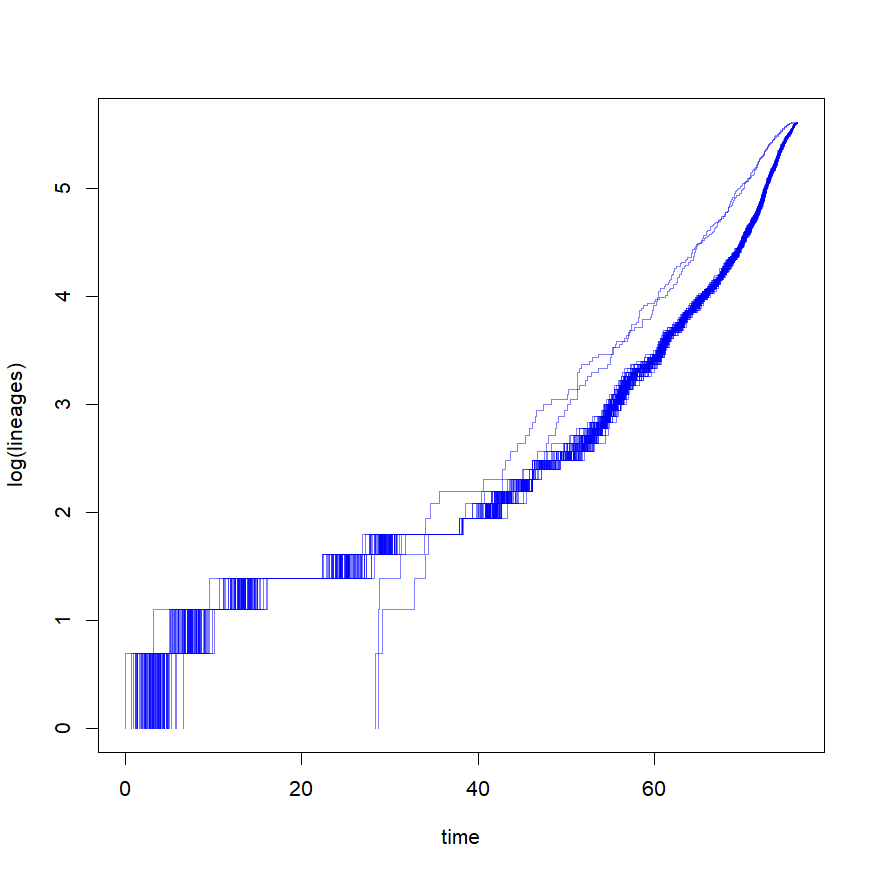Neat.

#### 1 comment:

1.Hi Liam, I wonder if there is an example of an LTT plot by a character's state. For instance, in fishes, two lines in a figure showing LTT for freshwater vs. marine.

Note: due to the very large amount of spam, all comments are now automatically submitted for moderation.